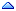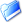GSI Forum
GSI Helmholtzzentrum für Schwerionenforschung

Home » BES3 » BES3 Physics » BES3 Physics Forum » How to get BES3 MC truth data?
Re: How to get BES3 MC truth data? [message #12414 is a reply to message #7894] Tue, 02 August 2011 11:10Cristina Morales Messages: 1Registered: May 2011 occasional visitor From: *kph.uni-mainz.de
Hi,

this is how I asked for the 4-momentum true values of my particles:
**********

SmartDataPtr<McParticleCol> mcParticles(eventSvc(),"/Event/MC/McParticleCol");
if( ! mcParticles ){
log << MSG::ERROR << " Unable to retrieve McParticleCol" << endreq;
return StatusCode::FAILURE;
}

for( McParticleCol::iterator it_mc = mcParticles->begin();
it_mc != mcParticles->end(); it_mc++ ){
cout<<"PID: "<<(*it_mc)->particleProperty()<<", px: "<<
(*it_mc)->initialFourMomentum().px()<<", py: "<<
(*it_mc)->initialFourMomentum().py()<<", pz: "<<
(*it_mc)->initialFourMomentum().pz()<<", E: "<<
(*it_mc)->initialFourMomentum().e()<<", theta: "
<<(*it_mc)->initialFourMomentum().theta()<<endl;

int PID = (*it_mc)->particleProperty();
double mcpx = (*it_mc)->initialFourMomentum().px();
double mcpy = (*it_mc)->initialFourMomentum().py();
double mcpz = (*it_mc)->initialFourMomentum().pz();
double mcE = (*it_mc)->initialFourMomentum().e();

m_mcPid = PID;
m_mcP4x = mcpx;
m_mcP4y = mcpy;
m_mcP4z = mcpz;
m_mcP4E = mcE;

m_mc->write();
}How to get BES3 MC truth data? By: Xia Wan on Tue, 17 February 2009 11:04Re: How to get BES3 MC truth data? By: Cristina Morales on Tue, 02 August 2011 11:10
Goto Forum:

Current Time: Tue Oct 26 21:18:25 CEST 2021

Total time taken to generate the page: 0.02514 seconds